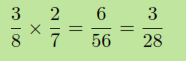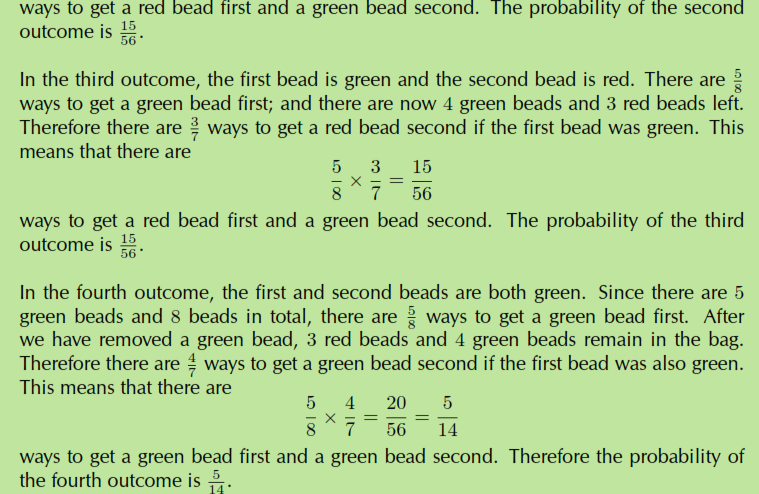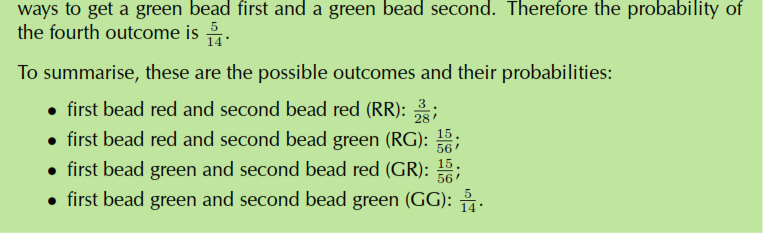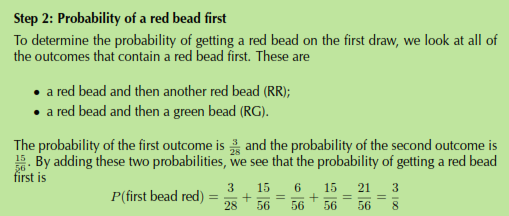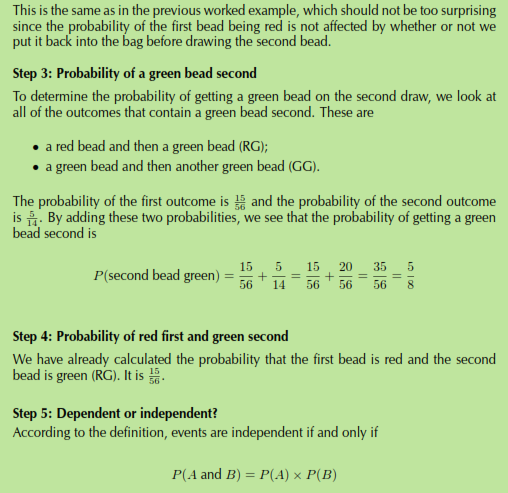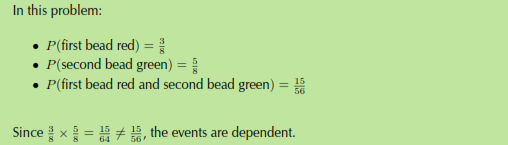Friday, March 24, 2023

#### 1. Revision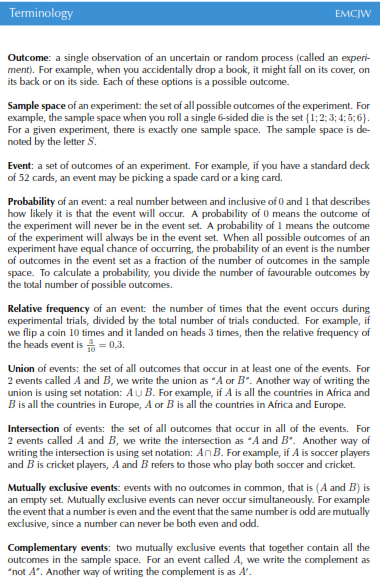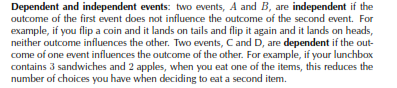#### 2. Identities

The addition rule (also called the sum rule) for any 2 events, A and B is

P(A or B) = P(A) + P(B) - P(A and B)

This rule relates the probabilities of 2 events with the probabilities of​​ their union and

intersection.

The addition rule for 2 mutually exclusive events is

P(A or B) = P(A) + P(B)

This rule is a special case of the previous rule. Because the events are mutually exclusive,

P(A and B) = 0.

The complementary rule is

P(not A) = 1​​ - P(A)

This rule is a special case of the previous rule. Since A and (not A) are complementary,

P(A or (not A)) = 1.

The product rule for independent events A and B is:

P(A and B) = P(A) x P(B)

If two events A and B are dependent then: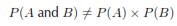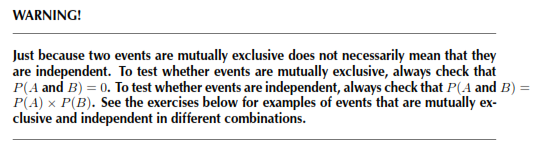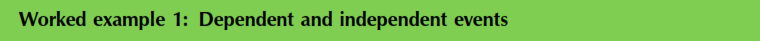QUESTION

Write​​ down which of the following events are dependent and which are independent:

1. The student council chooses a head student and then a deputy head student.

2. A bag contains blue marbles and red marbles. You take a red marble out of the​​ bag and then throw it back in again before you take another marble out of the bag.

SOLUTION

Step 1: Ask the question: Did the available choices change for the second event

because of the ﬁrst event?

1. Yes, because after selecting the head student there are fewer council​​ members available to choose for the deputy head student position. Therefore, the two events are dependent.

2. No, because when you throw the ﬁrst marble back into the bag, there are the

same number and color composition of choices for the second marble. Therefore

the two events are independent.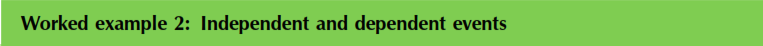QUESTION

A bag contains 3 yellow and 4 black beads. We remove a random bead from the bag,

record its colour and put it back into the bag. We then remove another random bead

from the bag and record its colour.

1.​​ What is the probability that the ﬁrst bead is yellow?

2. What is the probability that the second bead is black?

3. What is the probability that the ﬁrst bead is yellow and the second bead is black?

4. Are the ﬁrst bead being yellow and the second bead being black independent

events?

SOLUTION

Step 1: Probability of a yellow bead ﬁrst

Since there is a total of 7 beads, of which 3 are yellow, the probability of getting a

Step 2: Probability of a black bead second

The​​ problem states that the ﬁrst bead is placed back into the bag before we take the

second bead. This means that when we draw the second bead, there are again a total

of 7 beads in the bag, of which 4 are black. Therefore the probability of drawing a

Step 3: Probability of yellow ﬁrst and black second

When drawing two beads from the bag, there are 4 possibilities. We can get

We want to know the probability of the second outcome, where we have to get a yellow bead ﬁrst. Since there are 3 yellow beads and 7 beads in total, there are ways to get a yellow bead ﬁrst.​​ Now we put the ﬁrst bead back, so there are again 3 yellow beads and 4 black beads in the bag. Therefore there are 4/7 ways to get a black bead second if the ﬁrst bead was yellow. This means that there are 3/7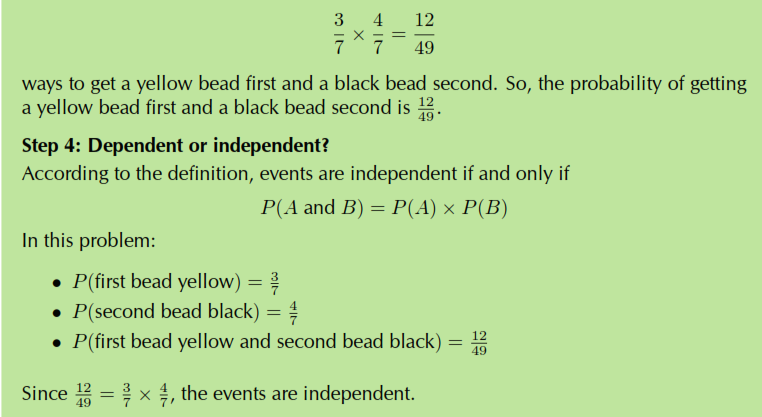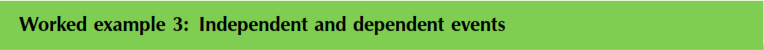QUESTION

In the previous example, we picked a random bead and put it back into the bag before continuing. This is called sampling with replacement. In this worked example, we will follow the same process, except that we will not put the ﬁrst bead back into the bag. This is called sampling without replacement. So, from a bag with 3 red and 5 green beads, we remove a random bead and record its colour. Then, without putting back the ﬁrst bead, we remove another random bead from the bag and record its colour.

1. What is the probability that the ﬁrst bead is red?

2. What is the probability that the second bead is green?

3. What is the probability that the ﬁrst bead is red and the second bead is green?

4. Are the ﬁrst bead being red and the second bead being green independent

events?

SOLUTION

Step 1:​​ Count the number of outcomes

We will examine the number ways in which we can get the different possible outcomes

when removing 2 beads. The possible outcomes are

For the ﬁrst outcome, we have to get a red bead ﬁrst. Since there are 3 red beads and

8 beads in total, there are​​ 3/8​​ ways to get a red bead ﬁrst. After we have taken out a red bead,​​ there are now 2 red beads and 5 green beads left. Therefore there are 2/7 ​​ ways to get a red bead second if the ﬁrst bead was also red. This means that there are​​